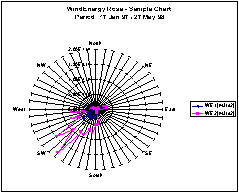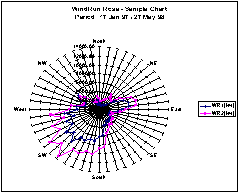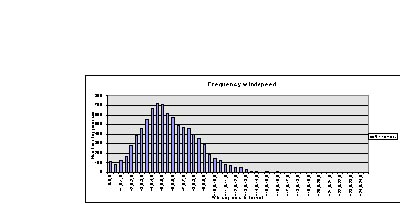DATA PROCESSING SERVICES & UTILITIES FOR WIND ENERGY APPLICATIONS

Clear and easy readable graphs are an essential tool in interpreting large quantities of data. Ekopower supplies several useful utilities for Excel in order to carry out quick and easy dataprocessing of data collected by the wind-meteo logger using
EKO21N or iBOX datalogger module.

Available software for data processing:-
- Basic wind utilities (running under Excel97)
- Dataprocessing services available (eg calculation wind energy production)

The advanced user is recommended to use both as some unique features
are available in that case.

#### The following basic wind utilities are available:• Convert data to roundhours
Normally the data is logged with a 10 minute interval, synchronized with real time clock. Meteo stations often record 1 hour average values. To carry out correlation with data from meteo-stations, the recorded 10 minute average values should be converted into 1 hour average values. By using the correlation and regression technique the long term behaviour of the local wind speed can be determined.

• Frequency distribution (graph & table)
Refer to sample graph below: red measured at the top of the mast and blue at a low level. The bin interval is selectable : 0.5 m/s or 1 m/s. Optional: calculation of the Weibull parameters which discribes the distribution mathematically.
• Windrun rose (graph & table)
Distribution of measured windrun (=total distance covered by the wind, like an odometer in a car). Refer to graph below.
• Windenergy rose (graph & table)
Distribution of available windenergy.Click on the graphs to enlarge

• Power curve (graph & table)
Each wind turbine has its own specific power curve: the power varies with the wind speed. This utility will create the typical power curve for the wind turbine, according to the latest recommendations. Included option: temperature and pressure will be taken into account.
• Energy output
It is important to calculate the energy output of a wind turbine, with known power curve, using the recorded frequency distribution. Normally the total energy output of a wind turbine is calculated during a one year period. This utility calculates energy ouput during the period of the wind speed measurements. Important for evaluation of different types of wind turbines for a specific site.
• Weibull parameter determination
• Correlation and regression analysis with other meteo stations in order to determine
the long term average wind speed, which is important in wind assessment studies.Frequency Distribution of wind speed